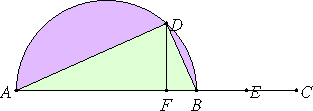# Proposition 34

To find two straight lines incommensurable in square which make the sum of the squares on them medial but the rectangle contained by them rational.

Set out two medial straight lines AB and BC, commensurable in square only, such that the rectangle which they contain is rational and the square on AB is greater than the square on BC by the square on a straight line incommensurable with AB.

Describe the semicircle ADB on AB. Apply a parallelogram to AB equal to the square on BE and deficient by a square figure, namely the rectangle AF by FB. Then AF is incommensurable in length with FB.Draw FD from F at right angles to AB, and join AD and DB.

X.11

Since AF is incommensurable in length with FB, therefore the rectangle BA by AF is also incommensurable with the rectangle AB by BF.

But the rectangle BA by AF equals the square on AD, and the rectangle AB by BF equals the square on DB, therefore the square on AD is also incommensurable with the square on DB.

And, since the square on AB is medial, therefore the sum of the squares on AD and DB is also medial.

And, since BC is double DF, therefore the rectangle AB by BC is also double the rectangle AB by FD.

X.6

But the rectangle AB by BC is rational, therefore the rectangle AB by FD is also rational.

X.32.Lemma

But the rectangle AB by FD equals the rectangle AD by DB, so that the rectangle AD by DB is also rational.

Therefore two straight lines AD and DB incommensurable in square have been found which make the sum of the squares on them medial, but the rectangle contained by them rational.

Q.E.D.

## Guide

This proposition is used in proposition X.40.